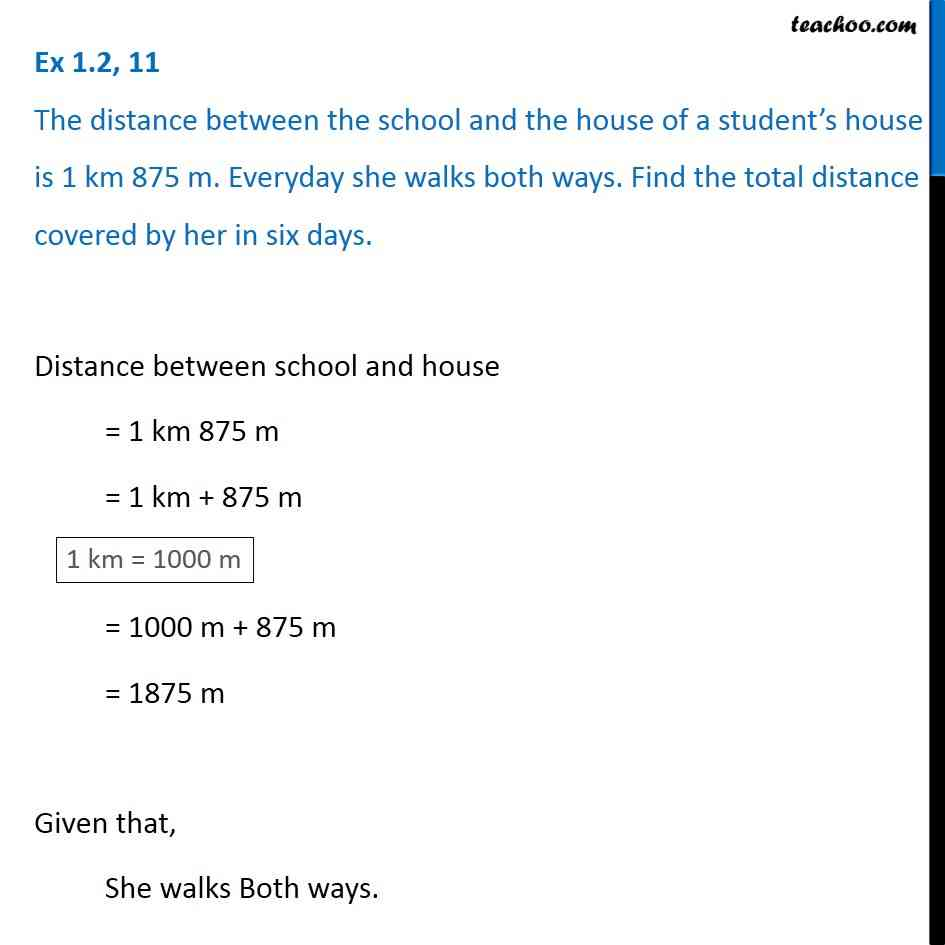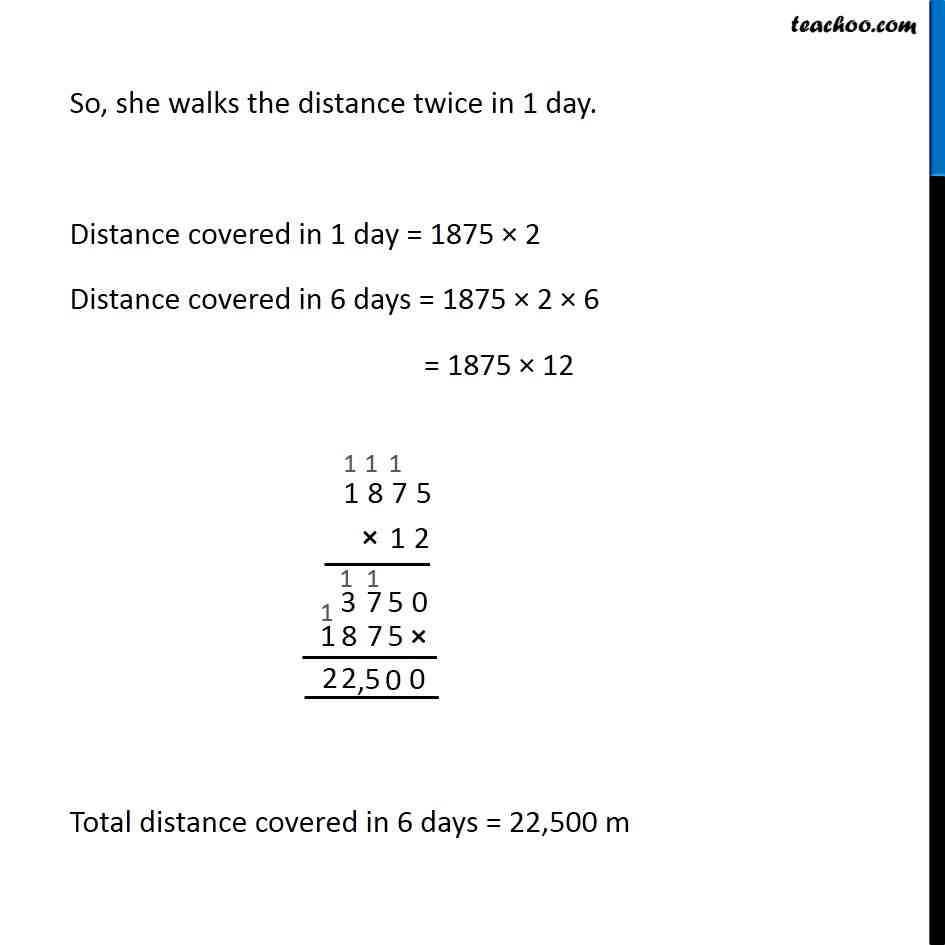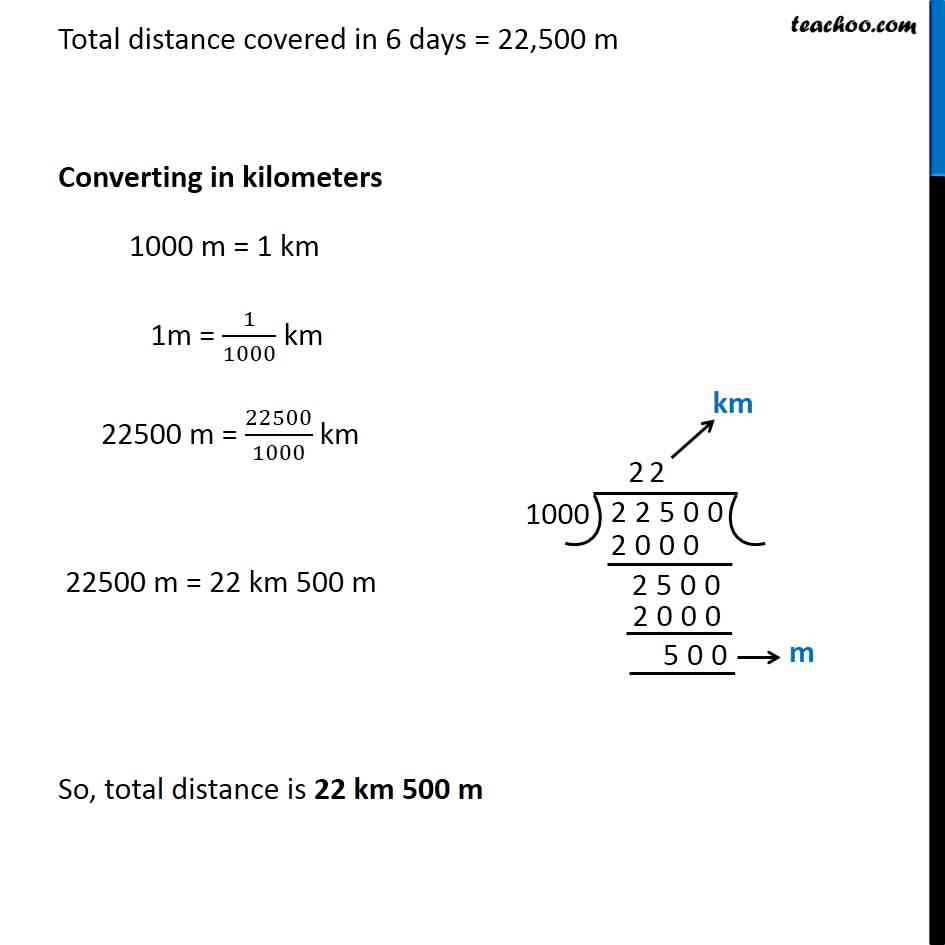Ex 1.2

Chapter 1 Class 6 Knowing our Numbers
Serial order wiseLearn in your speed, with individual attention - Teachoo Maths 1-on-1 Class

### Transcript

Ex 1.2, 11 The distance between the school and the house of a student s house is 1 km 875 m. Everyday she walks both ways. Find the total distance covered by her in six days.Distance between school and house = 1 km 875 m = 1 km + 875 m = 1000 m + 875 m = 1875 m Given that, She walks Both ways. So, she walks the distance twice in 1 day. Distance covered in 1 day = 1875 2 Distance covered in 6 days = 1875 2 6 = 1875 12 Total distance covered in 6 days = 22,500 m Total distance covered in 6 days = 22,500 m Converting in kilometers 1000 m = 1 km 1m = 1/1000 km 22500 m = 22500/1000 km 22500 m = 22 km 500 m So, total distance is 22 km 500 m